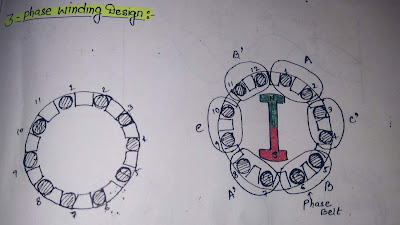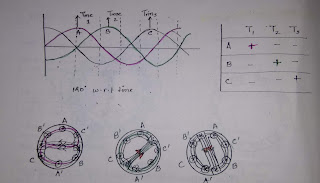#### 12 slot stator

Slot angle α = 180/slot/pole

Slot angle is 360°/12 = 30°3 Phase Stator winding Design

#### Phase spread (nα):-                                                                                                                     The total angle subtended by a phase group generally there are two phase spread for 3-⌀ winding , 120 and 60° phase. 60° phase spread is prcfered practically as it produce more voltage and more power rating comparatively.Winding Connection

• Here we can observe the supply voltage is moving w.r.t. time and also the M.F. is moving w.r.t. space which is called synchronous speed.3 Phase winding phase Difference

### Distribution factor or Breadth factor :-

Distribution Factor or the Breadth Factor is the ratio of the actual voltage obtained to the possible voltage if all the coils of a polar group were concentrated in a single slot. It's denoted by Kd.

Kd60/Kd120 =1.15

kd60 >kd120 hence phase spread of 60 is most prefered.
kd:- It is the factor by which the voltage is reduced when conductor are distributed.

n: slots/pole/phase

#### Slots/phase:- Always integer

Integer ⤗ integral slot winding
Non integer Fractional slot winding

The stator winding may be star or delta depending on starting method used (Refer starting method).